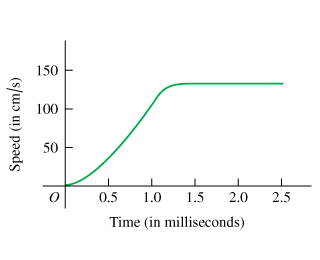# Problem: High-speed motion pictures ( 3500 ) of a jumping, 210 mu { m{g}} flea yielded the data used to plot the graph given in the figure . (See "The Flying Leap of the Flea" by M. Rothschild, Y. Schlein, K. Parker, C. Neville, and S. Sternberg in the November 1973 Scientific American.) This flea was about 2 mm long and jumped at a nearly vertical take-off angle. Use the graph to answer the questions.Is the acceleration of the flea ever zero? If so, when? Justify your answer.Find the maximum height the flea reached in the first 2.5 ms.Find the fleas acceleration at 0.5 ms.Find the fleas height at 0.5 ms.Find the fleas acceleration at 1.0 ms.Find the fleas acceleration at 1.5 ms.Find the fleas height at 1.0 ms.Find the fleas height at 1.5 ms.

⚠️Our tutors found the solution shown to be helpful for the problem you're searching for. We don't have the exact solution yet.

###### Problem Details

High-speed motion pictures ( 3500 ) of a jumping, 210 flea yielded the data used to plot the graph given in the figure. (See "The Flying Leap of the Flea" by M. Rothschild, Y. Schlein, K. Parker, C. Neville, and S. Sternberg in the November 1973 Scientific American.) This flea was about 2 mm long and jumped at a nearly vertical take-off angle. Use the graph to answer the questions.

Is the acceleration of the flea ever zero? If so, when? Justify your answer.

Find the maximum height the flea reached in the first 2.5 ms.

Find the fleas acceleration at 0.5 ms.

Find the fleas height at 0.5 ms.

Find the fleas acceleration at 1.0 ms.

Find the fleas acceleration at 1.5 ms.

Find the fleas height at 1.0 ms.

Find the fleas height at 1.5 ms.